Mobile QR CodeTemperature sensor, diode sensor, Temperature sensitivity, diode voltage, diode current

## I. INTRODUCTION

Temperature sensors are being widely used in advancing technology for various applications in semiconductor, automobile, aviation, environmental fields and so on. Until now, many types of temperature sensors have mainly been studied and used such as thermocouples, thermistors, resistance temperature detectors (RTDs), infrared temperature sensors, and the like. In the case of thermocouples, it is possible to measure over a wide temperature range, but the accuracy and stability are low. Thermistors have better accuracy than other types of sensors, but their temperature measurement range is limited. RTDs are stable over a limited temperature range, but have a narrow temperature measurement range. Besides, RTDs are expensive, and measurement inaccuracy occurs due to self-heating. For infrared temperature sensors, they can measure temperature without contact, even at high temperatures. However, they are sensitive to surface radiation with low accuracy.

A diode, a type of semiconductor device, is also being researched as a temperature measuring device. The range of use of semiconductor temperature sensors including diodes is about -50 degrees to +150 degrees, which is wider than other types of temperature sensors except for thermocouple temperature sensors. A diode temperature sensor is a device that uses the temperature dependency of the forward voltage of the diode terminals. As the temperature rises, the diode terminal voltage exhibits a characteristic of about -1 to -2 $\mathrm{mV} /{ }^{\circ} \mathrm{C}$. The previous studies using diodes as temperature sensors lack compatibility. They proposed a system that is only applicable to the particular type of diode used in their studies. In addition, complex and massive conditioning circuits with multiple op-amps, BJTs, diodes were used to implement semiconductor temperature sensor systems ((1-8)), increasing system volume and cost. Furthermore, in previous studies, the sensitivity before the conditioning circuit depends on the sensitivity of the diode itself, and the sensitivity of the diode is regarded as a constant with respect to temperature change. On the other hand, in this study, we prove that the temperature sensitivity of the diode voltage is not constant, and in order to increase the accuracy of the temperature sensitivity of the diode voltage, the rate of change of the temperature sensitivity of the diode voltage for temperature change (2nd derivative of the temperature of the diode voltage) is newly introduced. In addition, in order to increase the temperature sensitivity of the entire system, using the voltage ratio between the diode and the resistor is proposed instead of using only the sensitivity of the diode itself. Also, a simple voltage source method is used in this study to reduce the complexity and volume of the system due to the implementation of the current source used in the most previous studies. Another advantage of this study is that the temperature sensitivity of the system can be controlled by adjusting the value of the applied voltage source, and the compatibility problem can be solved simply by the reference and measured values with single calibration point regardless of the type of diode used. It is a great solution for flexible and low-cost applications.

## II. Theories

The output of diodes used as temperature sensors is the diode voltage $\left(V_{D}\right)$. Temperature curve of $V_{D}$ is pretty linear at least over the small region. That is around $0.5 \sim 0.8 \mathrm{~V}$ depending on the bias conditions. The theoretical equation for the diode current $\left(I_{D}\right)$ can be shown to be

##### (1)
\begin{equation} I_{D}=I_{S}\left(e^{\frac{V_{D}}{\eta V_{T}}}- 1\right) \end{equation}

where $I_{S}$ is the saturation current, $\eta$ is the emission coefficient, $V_{T}(=k T / q)$ is the thermal voltage, and $T$ is the absolute temperature. Note that the maximum average forward rectified current of diode is normally limited to $1 A$. As shown in Fig. 1, the diode has the temperature-dependent voltage-current characteristics. As the temperature increases, the diode voltage tends to decrease. In the following, we'll discuss why it decreases and how much it decreases with vary ing temperatures.

Through many mathematical processes from Eq. (1), the expression for temperature sensitivity of diode voltage $\left(s \equiv d V_{D} / d T\right)$ is given by

Fig. 1. The simulation results for diode voltage ($V_{D}$) and diode current ($I_{D}$) characteristics with varying temperatures and its circuit diagram.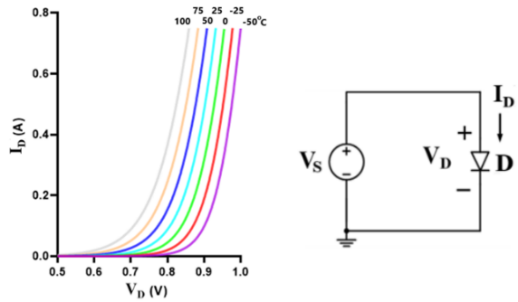##### (2)
\begin{equation} s=\frac{dV_{D}}{dT}=\frac{V_{D}- 3V_{T}- V_{G0}}{T}\Rightarrow s_{0}=\frac{V_{D0}- 3V_{T}- V_{G0}}{T_{0}} \end{equation}

Note that Eq. (2) is regardless of ƞ. $V_{G0}$ (= $E_{G0}$/q) and $E_{G0}$ is the semiconductor bandgap voltage and bandgap energy at the reference temperature $T_{0 }$($T$ = 298$K$), respectively. For silicon, $E_{G0}$ = 1.12 $eV$ and, therefore, $V_{G0}$ = 1.12 $V$. Also, note that $E_{G}$ can be assumed to be constant with temperature albeit it’s not a constant because its effect on the sensitivity is negligible. In Eq. (2), we can find that $s$ is always negative and therefore, $V_{D}$ drops with increasing $T$.

### 1. Temperature Sensitivity

The sensitivity, $s$, can be obtained using Eq. (2) once $V_{D}$ is given at the reference temperature. The prediction is in good agreement with the result from simulation. For example, $V_{D0 }$= 0.6867 $V$ (at $T$ = 298 $K$ and $I_{D }$= 10 $mA$), yields $s_{0 }$= (0.6867-3${\cdot}$0.02568-1.11)/298 = -1.678 $mV^{\circ}$C in Eq. (2) whereas $s_{0}$ = -1.680 $mV^{\circ}$C from simulation results. Note that we need to check $E_{G0}$ value prior to calculation (e.g., in the simulation, $E_{G0}$ = 1.11 $eV$ for 1N4002 diode) and subscript $_{\mathrm{‘}}$$_{0}$$_{\mathrm{’}}$ denotes the value of any parameter at the reference temperature $T_{0}$. Note that $T$ has units of $K$ and $T$ is 298 at room temperature.

### 2. Temperature Prediction

Once we have $V_{D0}$ and $s_{0}$, we can rewrite Eq. (2)

##### (3)
\begin{equation} V_{D}=V_{D0}+s_{0}\Delta T \end{equation}

where ${\Delta}$$T(=T_{m}-T_{0}) is the difference between measurement T (=T_{m}) and the reference T (=T_{0}). In order to predict T more accurately, we adopt the notation A as below ##### (4) \begin{equation} A=\frac{ds}{dT}=\frac{- 3V_{T}}{T^{2}}\Rightarrow A_{0}=\frac{- 3V_{T}}{{T_{0}}^{2}} \end{equation} Note that A is not constant versus temperature. And, A$$_{0}$ is the value of $A$ at the reference temperature $T_{0}$. If we denote the average of $A$ as $\overline{A}~ ($over {\Delta}T from T_{0}), ##### (5) \begin{align} \begin{array}{l} \overline{A}=\frac{1}{\Delta T}\int _{T_{0}}^{T_{0}+\Delta T}A dT=\frac{1}{\Delta T}\int _{T_{0}}^{T_{0}+\Delta T}- \frac{3V_{T}}{T^{2}}dT\\ =\frac{1}{\Delta T}\left(\frac{- 3k}{q}\right)\ln \left(1+\frac{\Delta T}{T_{0}}\right)\cong - \frac{3V_{T}}{T^{2}}\left[1- \frac{\Delta T}{2T_{0}}- \frac{\left(\Delta T\right)^{2}}{2{T_{0}}^{2}}\right]\\ \cong - \frac{3V_{T}}{{T_{0}}^{2}}\left(1- \frac{\Delta T}{2T_{0}}\right)=A_{0}\left(1- \frac{\Delta T}{2T_{0}}\right) \end{array} \end{align} In Table 1(a), we present the equations for s, \overline{s}(= average s over {\Delta}T interval), and $V_{D}$. By using these relations, we can predict the temperature. Also, we can check how much the predicted values of $T$ match with those of the simulations. To do this, we have extensively simulated $V_{D}$ over temperature for constant $I_{D}$. The simulation circuit diagram is depicted in Fig. 2. In Table 1(a), we introduce $I$, $II$, and $III$ as a method to predict temperature. In Method I, we assume that $s$ is constant over the full temperature range, like in previous studies. Hence, the constant $s_{0}$, the value of $s$ at $T_{0}$, is used. Then, we adopt $A$$_{0} to reflect the temperature-dependent characteristic of s in Method II where A is assumed to be constant over {\Delta}$$T$ interval. On the other hand, in order to predict temperature more accurately, Method III reflects the average $A$ over ${\Delta}$$T interval as presented in Eq. (5) because A is not constant versus temperature as shown in Eq. (4). Three methods (I, II, and III) are presented for comparison of the accuracy of T prediction. For temperature measurements with small {\Delta}$$T$, there is no significant difference among 3 methods, but for large ${\Delta}$$T, Method II or III should be used for precise prediction. As shown in Table 1(a), the expression for V_{D} must reflect the average s(=\overline{s}) over {\Delta}$$T$ interval to predict temperature. That is, $V_{D}=V_{D0}+\overline{s}\Delta T$. Note that $s$ and $A$ is always negative, as can be seen in Eqs. (2) and (4), respectively. The expressions for sensitivity $s$ in Table 1(a) show the fact that the magnitude of $s$ increases with rising temperatures. Therefore, as shown in Table 1(c), assuming a constant $s$ (= $s_{0}$) yields a bigger ${\Delta}$$T prediction in magnitude at high temperatures, whereas a smaller {\Delta}$$T$ prediction in magnitude at low temperatures.

So, if $V_{D}$ changes versus $T$ is plotted, $V_{D}$ decreases slightly more at high temperatures but increases slightly less at low temperatures. The shape of the graph would be less linear especially at very high or low temperatures relative to the reference temperature. In Table 1(b), we present and compare the predicted values of $s$ and $V_{D}$ at a given temperature. The sensitivity $s$ at a certain temperature was obtained as a trend line formed by neighboring 10 data (1 data per degree) around that temperature and it was very close to the results from Eq. (2), as expected. Table 1(c) presents the predicted values of $T$ in 25 degree increments at temperatures ranging from -50 to 100$^{\circ}$C only for Method I and $II$. The simulation results of $V_{D}$ and $V_{D0}$ are only used for prediction. It is observed that the prediction values agree very precisely using $Method II$ and $III$, even $I$. It can be concluded that the temperature prediction is sufficiently accurate even with Method II, especially true in Method III.

Note that $\Delta T$ is so simple and easy to calculate for Method $I$ where $\Delta T=\left(V_{D}-V_{D 0}\right) / s_{0}$. However, we need to solve the quadratic equation of $\Delta T$ in Method II and it is given by

##### (6)
\begin{equation} \Delta T=\left[- s_{0}- \sqrt{s_{0}^{2}+2A_{0}\left(V_{D}- V_{D0}\right)}\right]/A_{0} \end{equation}

Note that $\left(V_{D}-V_{D 0}\right)$ can be rewritten as $\Delta V_{D}$. Furthermore, in Method III, to avoid solving the cubic equation of $\Delta T$, we may borrow the result from Method II and then some iterations to obtain a better estimate for $\Delta T$. If the values are very close to the values from the previous iteration, no further iterations are necessary.

Fig. 3. The simulation circuit diagram of $V_{R}$/$V_{D}$ approach and its experimental setup.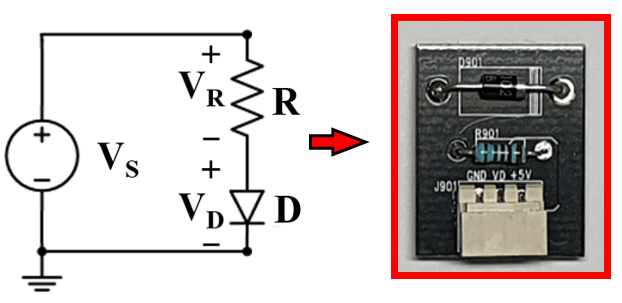## III. System design and Analysis

In order to increase $s$, we propose the ($V_{R}$/$V_{D}$) approach, in which we use a single resistor and diode. The circuit diagram for the $V_{R}$/$V_{D}$ simulation and its experimental setup is presented in Fig. 3.

For $V_{R}$/$V_{D}$ calculation,

##### (7)
\begin{equation} V_{D}=V_{D0}+\overline{s}\Delta T, V_{R}=V_{R0}- \overline{s}\Delta T \end{equation}

where $\overline{s}$ is the average $s$ over {\Delta}T interval. Then, ##### (8) \begin{align} \begin{array}{l} \frac{d}{dT}\left(\frac{V_{R}}{V_{D}}\right)=\frac{d}{dT}\left(\frac{V_{R0}- \overline{s}\Delta T}{V_{D0}+\overline{s}\Delta T}\right)=\frac{d}{dT}\left(\frac{V_{R0}\left(1- \frac{\overline{s}\Delta T}{V_{R0}}\right)}{V_{D0}\left(1+\frac{\overline{s}\Delta T}{V_{D0}}\right)}\right)\\ \cong \frac{d}{dT}\left[\frac{V_{R0}}{V_{D0}}\left(1- \frac{\overline{s}\Delta T}{V_{R0}}\right)\left(1- \frac{\overline{s}\Delta T}{V_{D0}}\right)\right] \end{array} \end{align} where we use an approximation 1 /[1+f(x)] \cong 1-f(x) which is especially true for |f(x)| <1. Once V_{D 0} is given, we can determine s_{0} and A_{0} in Eqs. (2) and (4), respectively. Also, $V_{R0}$ = $V_{S}$ - V_{D0}_{\mathrm{.}} Now, we’ll apply the Method I in which s=\overline{s}=s_{0}, as explained earlier. Assuming \overline{s}= s_{0} in Eqs. (7) and (8) leads to ##### (9a) \begin{align*} \begin{array}{l} \frac{d}{dT}\left(\frac{V_{R}}{V_{D}}\right)=\frac{d}{dT}\left\{\frac{V_{R0}}{V_{D0}}\left[1- \left(\frac{1}{V_{D0}}+\frac{1}{V_{R0}}\right)s_{0}\Delta T+\frac{s_{0}^{2}\left(\Delta T\right)^{2}}{V_{D0}V_{R0}}\right]\right\}\\ =\frac{d}{dT}\left[- \frac{s_{0}V_{S}\Delta T}{V_{D0}^{2}}+\frac{s_{0}^{2}\left(\Delta T\right)^{2}}{V_{D0}^{2}}\right]=- \frac{s_{0}V_{S}}{V_{D0}^{2}}+\frac{2s_{0}^{2}\Delta T}{V_{D0}^{2}} \end{array} \end{align*} where 2s_{0}^{2}\Delta T is relatively small compared to -s_{0}V_{S} in magnitude because $s_{0}$ is generally in the range of about -1 to -2 $mV^{\circ}$C. Therefore, 2$s_{0}^{2}\Delta T$can be ignored as long as ${\Delta}$$T is not so big. Then, Eq. (9a) can be simplified to Table 2. Temperature sensitivity of V_{R}/V_{D} and {\Delta}$$T$ expressions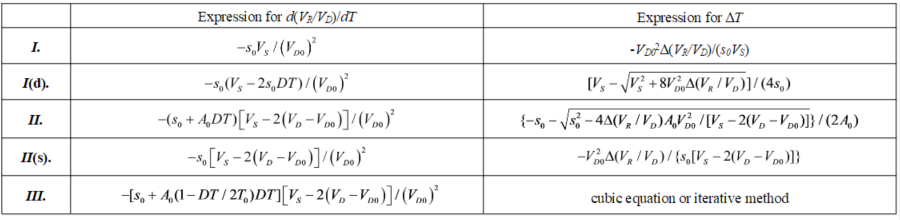##### (9b)
$\frac{d}{dT}(\frac{V_{R}}{V_{D}})=\frac{- s_{0}V_{S}}{V_{D0}^{2}}$

##### (12)
\begin{equation*} \frac{d}{dT}\left(\frac{V_{R}}{V_{D}}\right)=\frac{- \left[V_{S}- 2\left(V_{D}- V_{D0}\right)\right]}{V_{D0}^{2}}\left[s_{0}+A_{0}\left(1- \frac{\Delta T}{2T_{0}}\right)\Delta T\right] \end{equation*}

In Table 2, we summarize the temperature sensitivity of $V_{R}$/$V_{D}$ for each method.

where $\Delta T$ expressions can be obtained by exploiting the fact that $d\left(V_{R} / V_{D}\right) / d T=\left(V_{R} / V_{D}\right) / \Delta T$. As an example, for Method $I$ (detailed), $\Delta T$ can be calculated by solving quadratic equation of $\Delta \mathrm{T}$ and it is given by

##### (13)
\begin{equation} \Delta T=\left[V_{S}- \sqrt{V_{S}^{2}+8V_{D0}^{2}\Delta \left(V_{R}/V_{D}\right)}\right]/\left(4s_{0}\right) \end{equation}

For the purpose of comparing temperature predictions, we have presented 5 Methods ($I$, $I$(d), $II$, $II$(s), $III$). The most accurate approach is Method III, which can be applied to a wider temperature range by considering the temperature-dependent characteristics of $s$ and $A$. However, the formula is very complex (Therefore, we’ll not mention Method III thereafter). Note that Method I and Method II(s) are easy to use. However, we may pick Method I and $II$ as the main prediction methods. Note that Method I is the simplest while Method II is most accurate. For small ${\Delta}$$T, we can use Method I for simple and quick calculations without significant errors. However, for large {\Delta}$$T$, Method II must be used for precise temperature measurement.

Fig. 4. Simulation results of (a) $V_{D}$, (b) $V_{R}$/$V_{D}$ (-25 ~75$^{\mathrm{ o}}$C).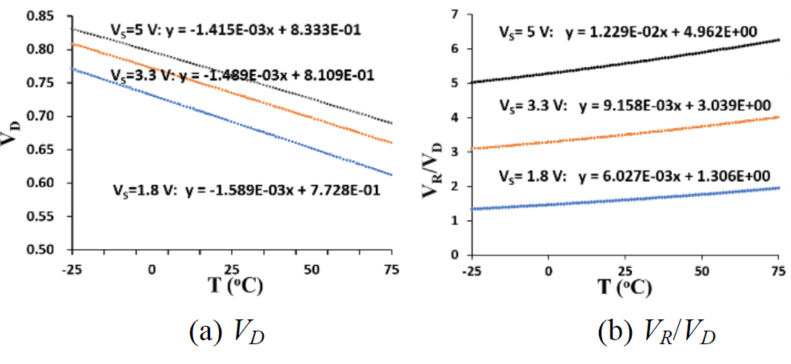Fig. 5. The plot of temperature prediction ($V_{S =}$ 1.8 $V$).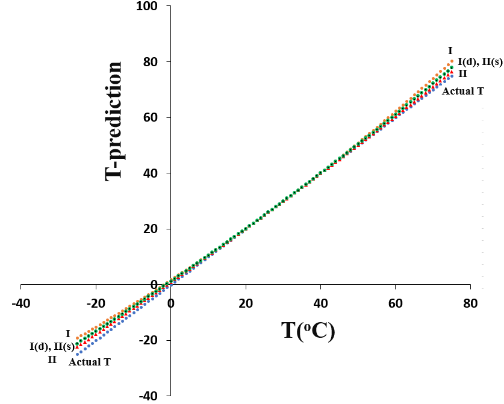In Fig. 4, we show the $V_{D}$ and $V_{R}$/$V_{D}$ simulation results for $V_{S =}$ 1.8, 3.3, and 5 $V$. All the plots appear to be linear over the whole temperature range (-25~75 $^{\mathrm{o}}$C). Here, the reference temperature $T_{0}$ = 25 $^{\mathrm{o}}$C, and the temperature range is 50 degrees in both directions from $T_{0 }$(${\Delta}$$T = {\pm} 50). Note that the temperature sensitivity prediction of V_{R}/V_{D} for the whole temperature range looks linear as shown in Fig. 4(b). In the simulation, the 1N4002 diode and 100 Ω resistor are used. However, for any single or discrete measurement with respect to T_{0}, the prediction gets worse as T_{m} deviates from T_{0}, especially for Method I. In addition, it is observed that the temperature sensitivity of V_{R}/V_{D} increases with V_{S}, as can be checked in Eqs. (9)-(12). Therefore, we can easily change the temperature sensitivity by controlling V_{S}. That is one of the main points of this study. We show the prediction temperature using V_{R}/V_{D} approach for V_{S =} 1.8 V, as plotted in Fig. 5. The reference temperature T_{0} is 25 ^{\mathrm{o}}C and the prediction near T_{0} is very accurate. However, temperature prediction gets worse as it deviates from the reference temperature. It can be clearly seen that the magnitude of {\Delta}$$T$ prediction is higher in the regions above $T_{0}$, whereas the magnitude of ${\Delta}$$T prediction is lower in the regions below T_{0}. It is because \overline{s}, as can be seen in Table 1(a), is always negative and more decreases (= large sensitivity in magnitude) with increasing temperatures. Since the approximation theory is applied in d(V_{R}/V_{D})/dT formula derivation processes, the exact {\Delta}$$T$ predictions are not possible especially when ${\Delta}$$T$ is large. As shown in Fig. 5, Method II appears to be the most accurate method close to the actual value.

## IV. Measurement Results

In Fig. 6, our temperature measurement setup is presented. A microprocessor-based temperature controller HSC-40 is used to monitor the temperature inside the chamber using a P-type thermocouple. The K-type GILTRON thermocouple (-200 ~ 200$^{\circ}$C) is additionally used to check the accurate temperature inside the chamber.

Note that $V_{D0}$ must be carefully measured prior to the temperature prediction. In order to utilize such diode circuits to measure temperature, one must obtain accurate values for $V_{D0}$ of the diode in advance.

The $V_{D }$values of experimental measurements, for $V_{S }$ = 1.8 $V$ and $R$ = 100 Ω, appear in Table 3. The graphs of $V_{D}$, $V_{R}$/$V_{D}$, and $T$ prediction are plotted in Fig. 7. Note that the simulation results agree well with the experimental results. For example, at 100 $^{\circ}$C, the temperature prediction error using Method II is less than 1 $^{\circ}$C.

Fig. 7. The experimental results for (a) $V_{D}$, (b) $V_{R} / V_{D=}$ (c) $T$ Prediction through $V_{R} / V_{D}$ approach using measurement values $\left(V_{S}=1.8 V\right.$ and $\left.R=100 \Omega\right)$.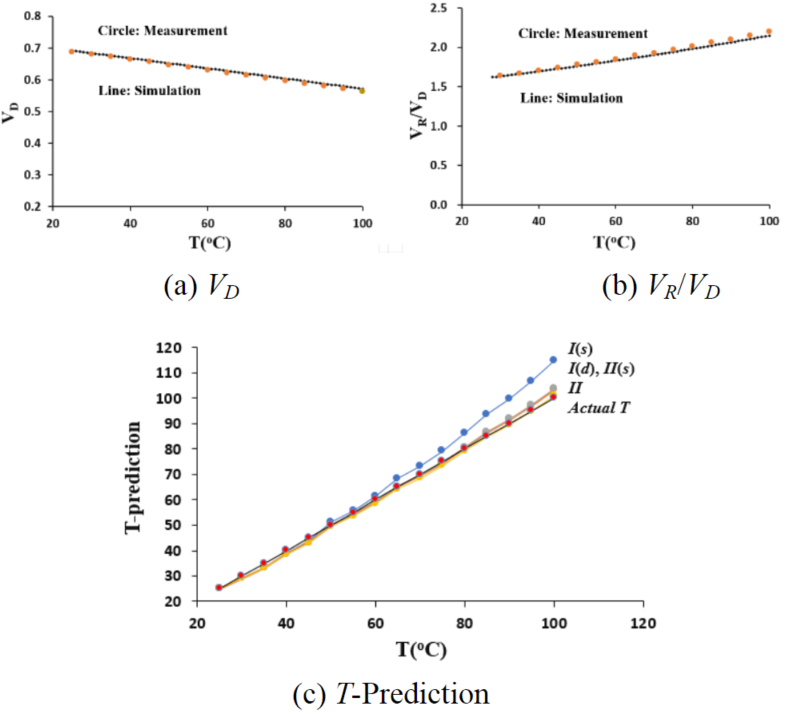## V. Conclusions

In this study, we derive the diode voltage-temperature relational equation. Then, in order to increase temperature sensitivity, we propose the ($V_{R}$/$V_{D}$) approach as a function of $V_{S}$ and $V_{D0}$, whose sensitivity is accurate over any small range of temperature with respect to the reference temperature. However, over the temperature ranges far from the reference, the modified equation must be used to reduce the error in estimation temperature. Also, by controlling $V_{s}$ for a specified $V_{D0}$, the temperature sensitivity can be increased by several times in magnitude (e.g., 8.7 times, $s$ = 12.3 $mV^{\circ}$C) compared to the typical 1N 4002 diode ($s$ = -1.42 $mV^{\circ}$C) for $V_{s}$ = 5.0 $V$, $R$ = 100 Ω, and $V_{D0}$ = 0.761 $V$. This work presents a diode temperature-measurement system with simplicity, high-sensitivity, and sensitivity-controllability.

### ACKNOWLEDGMENTS

This work was supported by the National Research Foundation of Korea (NRF) grant funded by the Korea government (MSIT) (No. 2019R1F1A1059031). Also, this work was supported by the National Research Foundation of Korea (NRF) (2019R1F1A1063151, 2021R1F1A1052193).

### REFERENCES

1
Mansoor M., Aug 2015, Silicon diode temperature sensors, Sens. & Actr., A: Phys., Vol. 232, No. 1, pp. 63-742
Dzinavatonga K., Dec 2016, Application of the 1N4148 Diode to temperature measurements between −140 °C and 5 °C, IEEE Sens. J., Vol. 16, No. 23, pp. 8542-85443
Rao S., Jan 2016, 4H-SiC p-i-n diode as Highly Linear Temperature Sensor, IEEE Trans. E. Dev., Vol. 63, No. 1, pp. 414-4184
Santra S., May 2010, Silicon on insulator diode temperature sensor-A detailed analysis for ultra-high temperature operation, IEEE Sens. J., Vol. 10, No. 5, pp. 997-10035
Precker J. W., Nov 2002, Experimental estimation of the bandgap in silicon and germanium from the temperature-voltage curve of diode thermometers, Am. J. Phys., Vol. 70, No. 11, pp. 1150-11536
Ocaya R. O., Apr 2006, An experiment to profile the voltage, current and temperature behaviour of a P-N diode, Eur. J. Phys., Vol. 27, No. 2006, pp. 625-6337
Sconza A., Jan 1994, Experiment on the physics of the PN junction, Am. J. of Phys., Vol. 62, No. 1, pp. 66-708
Ocaya R. O., Jan 2013, A linear, wide-range absolute temperature thermometer using a novel p-n diode sensing technique, Meas., Vol. 46, No. 2013, pp. 1464-1469## Author

Chun-Hyung Cho received the B.S. degree in Electrical Engineering from the Seoul National University, Seoul, South Korea, in 1997, and the M.S. and Ph.D. degrees in Electrical and Computer Engineering from Auburn University, Auburn, AL, in 2001 and 2007, respectively.

In 2009, he joined Hongik University, Sejong where he is a professor in the Department of Electronic & Electrical Convergence engineering.

His research interests include the application of analytical and experimental methods of piezoresistive sensors to problems in electronic packaging.

Hyuntai Kim obtained his Ph.D. degree from the School of Electrical Engineering, Seoul National Univer-sity (SNU), Korea, in 2016.

He subsequently held one-year post-doctoral fellowship at SNU.

He joined the Optoelectronics Research Centre (ORC) at the University of Southampton as a Research Fellow in 2017.

Since 2019, he has been with the Department of Electronic and Electrical Convergence Engineering, Hongik University as a Faculty Member (Assistant Professor).

He is primarily interested in fiber optics and nanophotonics.